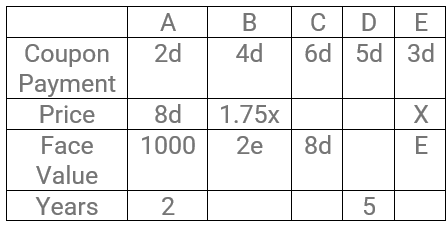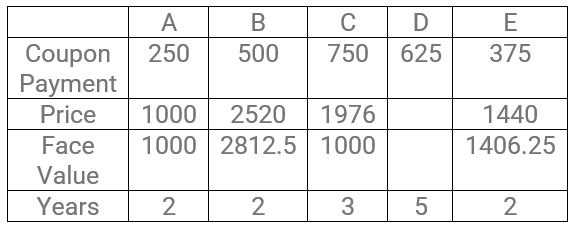### XAT 2010 Question 92

Instructions

Answer the questions based on the information given below:

Madhubala Devi, who works as a domestic help, received Rs. 2500 as Deepawali bonus from her employer. With that money she is contemplating purchase of one or more among 5 available government bonds - A, B, C, D and E.

To purchase a bond Madhubala Devi will have to pay the price of the bond. If she owns a bond she receives a stipulated amount of money every year (which is termed as the coupon payment) till the maturity of the bond. At the maturity of the bond she also receives the face value of the bond.
Price of a bond is given by: $$P=[\sum_{t=1}^T\frac{C}{(1+r)^{t}}]+\frac{F}{(1+r)^{t}}$$

where C is coupon payment on the bond. which is the amount of money the holder of the bond receives annually; F is the face value of the bond, which is the amount of money the holder of the bond receives when the bond matures (over and above the coupon payment for the year of maturity); T is the number of years in which the bond matures;
R = 0.25, which means the market rate of interest is 25%.

Among the 5 bonds the bond A and another two bonds mature in 2 years, one of the bonds matures in 3 years, and the bond D matures in 5 years.

The coupon payments on bonds A, E, B, D and C are in arithmetic progression, such that the coupon payment on bond A is twice the common difference, and the coupon payment on bond B is half the price of bond A.

The face value of bond B is twice the face value of bond E, but the price of bond B is 75% more than the price of bond E. The price of bond C is more than Rs. 1800 and its face value is same as the price of bond A. The face value of bond A is Rs. 1000.

Bond D has the largest face value among the five bonds.

Question 92

# Madhubala Devi purchased one or more of the 5 available bonds from her bonus pay and spent the remainder. She made the purchase decision such thather return from the bonds is maximized. Her returnfrom the bonds is

Solution

Let the coupon payments of A, E, B, D and C be a-2d, a-d, a, a+d, and a+2d, respectively.

Since, the coupon payment of A is twice the common difference,

a - 2d = 2d

a = 4d

Thus, the coupon payments of A, E, B, D and C are 2d, 3d, 4d, 5d, and 6d, respectively.

Since the coupon payment on bond B is half the price of bond A, the price of bond A = 2 x 4d = 8d.

Let the price of bond E be $$x$$. Thus, the price of bond B = $$1.75x$$.

Let the face value of bond E be $$e$$. Thus, the face value of bond B = $$2e$$.

Since the face value of bond C is equal to the price of bond A, the face value of C is 8d.

We know that three of the bonds mature in two years, two in three years and one in five years. We are also given that A matures in 2 and D matures in 5 years.

Tabulating the given information.Applying the price formula for A, we get

8d = $$\frac{2d}{1.25}+\frac{2d}{1.25^2}+\frac{1000}{1.25^2}$$

Solving this, we get $$d=125$$

Thus, the coupon payments of A, B, C, D, and E are 125, 500, 750, 625 and 375, respectively.

Price of A = 8d = 1000 = Face value of C

We are given that the price of bond C is more than 1800. The time period for C could be 2 or 3 years.

Case 1: Time period is 2 years.

Applying the price formula for C.

Price = $$\frac{750}{1.25}+\frac{750}{1.25^2}+\frac{1000}{1.25^2}$$

Price = 1720 (<1800)

Thus, the maturity period of bond C is 3 years and for bonds B and E will be 2 years.

Applying the price formula again, we get

Price = $$\frac{750}{1.25}+\frac{750}{1.25^2}+\frac{750}{1.25^3}+\frac{1000}{1.25^3}$$

Price of C = 1976

Using the price formula on bond B, we get

1.75$$x$$ = $$\frac{500}{1.25}+\frac{500}{1.25^2}+\frac{2e}{1.25^2}$$ ...(1)

Using the price formula on bond E, we get

$$x$$ = $$\frac{375}{1.25}+\frac{375}{1.25^2}+\frac{2}{1.25^2}$$ ...(2)

Solving equations (1) and (2) simultaneously, we get

$$x=1440$$ and $$e=1406.25$$

Thus, the table becomesSince Madhubala has Rs. 2500, she can either buy one bond each of A and E, or a single bond C.

Return from bonds A and E = 1000 + 2(250) + 1406.25 + 2(375) = 3656.25

Return from bond C = 1000 + 3(750) = 3250

Thus, the maximum return that Madhubala can get is Rs. 3656.25.

Hence, the answer is option C.